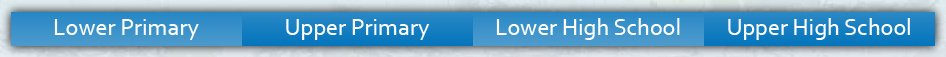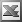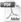Upper Primary Enter keywords to search by topic or worksheet description

Worksheets in this category help students to read, write and understand decimals, and to understand their relationship to both common fractions and percentages.

Worksheet Name Files
Decimals in Different Forms: Pictures, Numerals, WordsDecimals in Different Forms: Numerals, Fractions, WordsDecimals: Writing in WordsDecimals: Arranging in OrderDecimals: Comparing Using SymbolsRounding DecimalsRounding Decimals: INTERACTIVEDecimals: Place Value of Digits to ThousandthsDecimals: Place Value and Decimal AdditionDecimals: Estimating Decimal AdditionTerminating and Recurring Decimals